SSC  >  Test: Solid Mechanics- 2

# Test: Solid Mechanics- 2

Test Description

## 20 Questions MCQ Test Mock Test Series of SSC JE Civil Engineering | Test: Solid Mechanics- 2

Test: Solid Mechanics- 2 for SSC 2023 is part of Mock Test Series of SSC JE Civil Engineering preparation. The Test: Solid Mechanics- 2 questions and answers have been prepared according to the SSC exam syllabus.The Test: Solid Mechanics- 2 MCQs are made for SSC 2023 Exam. Find important definitions, questions, notes, meanings, examples, exercises, MCQs and online tests for Test: Solid Mechanics- 2 below.
Solutions of Test: Solid Mechanics- 2 questions in English are available as part of our Mock Test Series of SSC JE Civil Engineering for SSC & Test: Solid Mechanics- 2 solutions in Hindi for Mock Test Series of SSC JE Civil Engineering course. Download more important topics, notes, lectures and mock test series for SSC Exam by signing up for free. Attempt Test: Solid Mechanics- 2 | 20 questions in 12 minutes | Mock test for SSC preparation | Free important questions MCQ to study Mock Test Series of SSC JE Civil Engineering for SSC Exam | Download free PDF with solutions
 1 Crore+ students have signed up on EduRev. Have you?
Test: Solid Mechanics- 2 - Question 1

### The maximum deflection due to a uniformly distributed load w/unit length over entire span of a cantilever of length L and of flexural rigidity EI, is

Test: Solid Mechanics- 2 - Question 2

### The equivalent length of a column of length L having one end fixed and the other end free is

Detailed Solution for Test: Solid Mechanics- 2 - Question 2

Effective length of columns under different end conditions are:

1. For both ends hinged: Le = L

2. One end fixed and another end free: Le = 2L

3. Both ends fixed =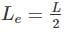4. One end fixed and other is hinges: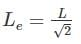Test: Solid Mechanics- 2 - Question 3

### Two bars of different materials are of the same size and are subjected to same tensile forces. If the bars have unit elongations in the ratio of 3 : 8, then the ratio of moduli of elasticity of the two materials is:

Test: Solid Mechanics- 2 - Question 4

The radius of Mohr’s circle for two equal and unlike principal stresses of magnitude “p” is:

Detailed Solution for Test: Solid Mechanics- 2 - Question 4

Radius of Mohr’s circle is given by: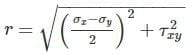At principal plane, σx = σ1, σy = σ2

τxy = 0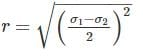σ= p , σ2 = -p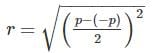r = p

Test: Solid Mechanics- 2 - Question 5

The correct relation between Modulus of elasticity (E), Shear Modulus (G) and Bulk modulus (K) is:

Detailed Solution for Test: Solid Mechanics- 2 - Question 5

Relation between Modulus of elasticity (E), Shear Modulus (G) and Bulk modulus (K) is given by: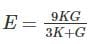Now, rewriting the equation as follows:

3KE + GE = 9KG

3KE – 9KG = - GE

K(3E – 9G) = - GE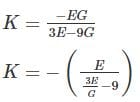Test: Solid Mechanics- 2 - Question 6

A simply supported beam with a gradually varying load from zero at B and w per unit length at A is shown in figure below, the shear force at B is equal to: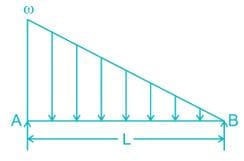Detailed Solution for Test: Solid Mechanics- 2 - Question 6

SFy = 0

RA + RB =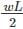Taking moment of all the forces about B = 0.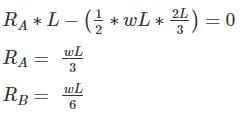Test: Solid Mechanics- 2 - Question 7

When the shear force diagram is a parabolic curve between two points, it indicates that there is a

Detailed Solution for Test: Solid Mechanics- 2 - Question 7

The general relationship between the shear force diagram, bending moment diagram and loading diagram will be:

2. Bending moment diagram is 1higher than shear force diagram.

3. For the uniformly varying load on the beam, the shear force diagram is parabolic in nature.

4. For the uniformly varying load on the beam, the bending moment diagram is also parabolic but is 1o higher than shear force diagram.

Test: Solid Mechanics- 2 - Question 8

The ratio of moment of inertia of circular plate to that of square plate of equal depth is:

Test: Solid Mechanics- 2 - Question 9

A circular shaft of 50 mm diameter is required to transmit a torque from one shaft to another. If shear stress is not to exceed 40 Mpa, the safe torque is:

Detailed Solution for Test: Solid Mechanics- 2 - Question 9

Using the relation: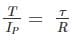Where,

T = Torque applied to the shaft

IP = Polar section modulus

τ = shear stress in the material

R = radius of the shaft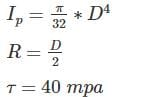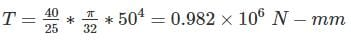= 0.982 kN-m

Test: Solid Mechanics- 2 - Question 10

Maximum Shear strain Energy theory was postulated by:

Detailed Solution for Test: Solid Mechanics- 2 - Question 10

1. Maximum Principal stress theory was postulated by Rankine. It is suitable for brittle materials.

2. Maximum Principal strain theory was postulated by St Venant. This theory is not accurate for brittle and ductile materials both.

3. Maximum Shear stress theory was postulated by Tresca. This theory is suitable for ductile materials. Its results are the safest.

4. Maximum shear strain energy theory was postulated by Von-mises. Its results in case of pure shear are the accurate for ductile materials.

Test: Solid Mechanics- 2 - Question 11

If a shaft turns at 150 rpm under a torque of 1500 Nm, then power transmitted is

Detailed Solution for Test: Solid Mechanics- 2 - Question 11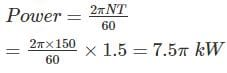Test: Solid Mechanics- 2 - Question 12

If a close coiled helical spring absorbs 30 N-mm of energy while extending by 5mm, its stiffness will be ______.​

Detailed Solution for Test: Solid Mechanics- 2 - Question 12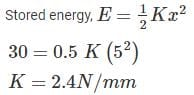Test: Solid Mechanics- 2 - Question 13

A steel bar of 5mm is heated from 5°C to 40°C and is free to expand. The bar will induce

Detailed Solution for Test: Solid Mechanics- 2 - Question 13

When a material undergoes a change in temperature, it either elongates or contracts depending upon whether temperature is increased or decreased of the material. If the elongation or contraction is not restricted, i. e. free then the material does not experience any stress despite the fact that it undergoes a strain.

Test: Solid Mechanics- 2 - Question 14

A bar of uniform thickness t and Length L tapers uniformly from a width of b1 at one end to b2 at the other end. What will be the extension of the bar under an axial pull P?

Test: Solid Mechanics- 2 - Question 15

The number of elastic constants for a completely anisotropic elastic material which follows Hooke’s law is

Detailed Solution for Test: Solid Mechanics- 2 - Question 15

Explanation : Non isotropic or anisotropic materials have different properties in different directions. They show non- homogeneous behaviour. The number of elastic constants is 21.

Test: Solid Mechanics- 2 - Question 16

The Poisson’s ratio of a material which has Young’s modulus of 120 GPa, and shear modulus of 50 GPa, is

Detailed Solution for Test: Solid Mechanics- 2 - Question 16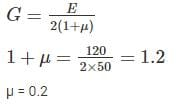Test: Solid Mechanics- 2 - Question 17

The change in the slope between two points in a straight member under flexure is equal to the area of M/EI diagram between those two points. This statement is known as -

Test: Solid Mechanics- 2 - Question 18

A simply supported beam is of rectangular section. It carries a uniformly distributed load over the whole span. The deflection at the centre is “y”. If the depth of the beam is doubled, the deflection at centre will be:

Detailed Solution for Test: Solid Mechanics- 2 - Question 18

Deflection of beam is inversely proportional to area moment of inertia of the beam.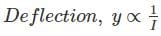Moment of inertia for rectangular section,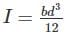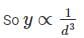Let the final deflection is yfinal when the depth is doubled.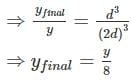Test: Solid Mechanics- 2 - Question 19

The ratio of the moment of resistance of a solid circular shaft of diameter D and a hollow shaft (external diameter D and internal diameter d) is given by

Detailed Solution for Test: Solid Mechanics- 2 - Question 19

As we know,

M ∝ Z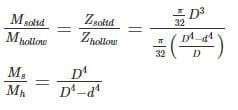Test: Solid Mechanics- 2 - Question 20

Rankine theory is applicable to the ________.

Detailed Solution for Test: Solid Mechanics- 2 - Question 20

For short or long columns Rankine’s Formula is used.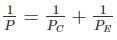Where P is crippling load by Rankine formula;  PC is crushing load; PE is crippling load by Euler’s formula.

For Short column: PE is very large so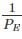will be very small and negligible as compared to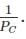so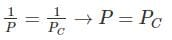For Long column: PE is small so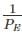will be large as compared to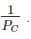Hence the value of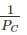can be neglected. So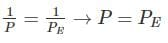Hence the crippling load by Rankine’s formula for long column is approximately equal to the crippling load by Euler’s formula.

## Mock Test Series of SSC JE Civil Engineering

1 videos|1 docs|64 tests
 Use Code STAYHOME200 and get INR 200 additional OFF Use Coupon Code
Information about Test: Solid Mechanics- 2 Page
In this test you can find the Exam questions for Test: Solid Mechanics- 2 solved & explained in the simplest way possible. Besides giving Questions and answers for Test: Solid Mechanics- 2, EduRev gives you an ample number of Online tests for practice

## Mock Test Series of SSC JE Civil Engineering

1 videos|1 docs|64 tests